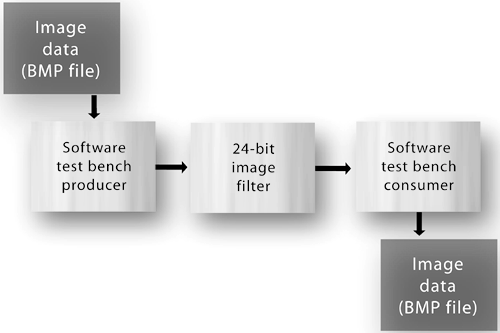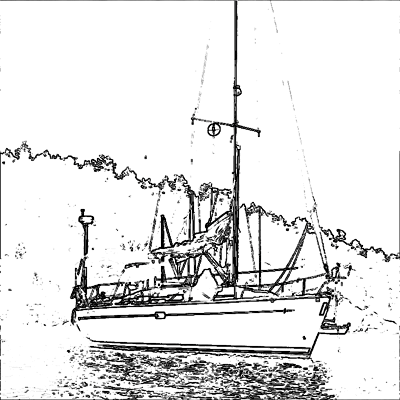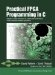# Section 11.1. Design Overview

### 11.1. Design Overview

Edge-detection algorithms are used to identify and enhance areas of an input image (whether a single image or a real-time video stream) that have particularly high contrast between adjacent pixels. There are many different edge-detection algorithms, each of which is optimized for particular requirements and for a particular hardware or software implementation.

Virtually all image-detection algorithms, and many other types of imaging filters, share a common attribute: they operate iteratively on specific "windows" of an image, where a window is defined as a collection of neighboring pixels spanning a number of rows and columns, with the target pixel in the center. In this example, we use a 3-by-3 window.

If we wish to create an image filter that operates on a stream of pixel data (see Figure 11-1), one pixel at a time, it is clear that the process must store at least enough pixel values to "look ahead" one row (plus one pixel) and "look behind" one row (plus one pixel). To do this, we will describe a circular streaming image buffer using some simple array indexing techniques in C:

` while ( co_stream_read(pixels_in, &nPixel, sizeof(co_uint24)) == co_err_none ) {           bytebuffer[addpos][REDINDEX] = nPixel & REDMASK;          bytebuffer[addpos][GREENINDEX] = (nPixel & GREENMASK) >> 8;          bytebuffer[addpos][BLUEINDEX] = (nPixel & BLUEMASK) >> 16;          addpos++;          if (addpos == BYTEBUFFERSIZE)                   addpos = 0;          currentpos++;          if (currentpos == BYTEBUFFERSIZE)                   currentpos = 0; `

##### Figure 11-1. A single-process edge-detection filter and test bench.The result of reading the 24-bit input data into the local storage area and unpacking the color values is three distinct buffers, one for each color, containing exactly enough pixel data at any given time to provide access to the required 3-by-3 window over a single scan line.

Once we have access to a window of pixel data, we can then perform the necessary calculations to determine the new value of the target pixel. We could make many possible calculations to produce the desired results. The edge-detection algorithm described in this example performs a relatively primitive set of calculations on the image window to enhance the edges: For each pixel n, four pairs of pixels, each pair surrounding n on one axis, are compared, and their absolute difference is calculated for each pixel color (red, green, and blue). For each color, a new value for n is assigned that represents the greatest observed difference between any enclosing pair of pixels.

This logic is expressed in an inner code loop (nested within a larger loop running over each target pixel), as shown in Figure 11-2. Let's examine this loop in detail:

##### Figure 11-2. Edge-detection processing loop for one pixel and three colors.
` for (clr = 0; clr < 3; clr++) {  // Red, Green and Blue    pixelN  = bytebuffer[B_OFFSETADD(currentpos,WIDTH)][clr];    pixelS  = bytebuffer[B_OFFSETSUB(currentpos,WIDTH)][clr];    pixelE  = bytebuffer[B_OFFSETADD(currentpos,1)][clr];    pixelW  = bytebuffer[B_OFFSETSUB(currentpos,1)][clr];    pixelNE = bytebuffer[B_OFFSETADD(currentpos,WIDTH+1)][clr];    pixelNW = bytebuffer[B_OFFSETADD(currentpos,WIDTH-1)][clr];    pixelSE = bytebuffer[B_OFFSETSUB(currentpos,WIDTH-1)][clr];    pixelSW = bytebuffer[B_OFFSETSUB(currentpos,WIDTH+1)][clr];    // Diagonal difference, lower right to upper left    pixelMag = 0;    pixeldiff = ABS(pixelSE - pixelNW);    if (pixeldiff > pixelMag)        pixelMag = pixeldiff;               // Diagonal difference, upper right to lower left    pixeldiff = ABS(pixelNE - pixelSW);    if (pixeldiff > pixelMag)        pixelMag = pixeldiff;    // Vertical difference, bottom to top    pixeldiff = ABS(pixelS - pixelN);    if (pixeldiff > pixelMag)        pixelMag = pixeldiff;    // Horizontal difference, right to left    pixeldiff = ABS(pixelE - pixelW);    if (pixeldiff > pixelMag)        pixelMag = pixeldiff;    if (pixelMag < EDGE_THRESHOLD)        pixelMag = 0;     nByteMag[clr] = (co_uint8) pixelMag; } `

• For each color (red, green, and blue) in the target pixel, the values for the eight adjacent pixels are copied from the scan line buffer (bytebuffer). Note that the B_OFFSETADD and B_OFFSETSUB macros are defined elsewhere (in an include file) and provide the circular buffer offsets needed to access the desired pixels.

• After the adjacent pixel values have been determined (and stored in local variables PixelN, PixelNE, PixelW, and so on), a set of four calculations is performed to determine the maximum difference in intensity between any two bounding pixelsfor example, between PixelN and PixelS. The absolute value operation, in the form of the ABS macro defined elsewhere in an include file, is used in these calculations.

• After all four possible edge directions (the vertical, the horizontal, and the two diagonals) have been analyzed, the maximum intensity difference is compared to the edge threshold value (EDGE_THRESHOLD). If it is greater than the threshold value, it becomes the new value of the pixel. If the difference does not meet the threshold value, the color is given a value of zero, which corresponds to black. (This calculation can be easily modified to produce different levels of contrast in the output image or to produce a black-on-white image, as shown in Figure 11-4.)

##### Figure 11-4. Image after edge-detection filtering.After the new values for the three colors are determined, they are repackaged into a single 24-bit value and written to the output buffer using co_stream_write:

` nPixel = nByteMag[REDINDEX] |     (nByteMag[GREENINDEX] << 8) |     (nByteMag[BLUEINDEX] << 16); co_stream_write(pixels_out, &nPixel, sizeof(co_uint24)); `

The result is a single image filter process that accepts 24-bit pixels, caches just enough pixels locally (using a circular array) to assemble a window of eight pixels around each of the pixels in the scan line of interest, splits these eight required pixels into their component colors, and performs the edge-detection function. As you will see, there are more efficient ways to implement a filter such as this, but as a starting point (perhaps as a working prototype) this filter will produce the desired outputs and can be compiled to hardware.Practical FPGA Programming in C
ISBN: 0131543180
EAN: 2147483647
Year: 2005
Pages: 208

Similar book on Amazon